Get instant live expert help with Excel or Google Sheets“My Excelchat expert helped me in less than 20 minutes, saving me what would have been 5 hours of work!”

#### Post your problem and you’ll get Expert help in seconds.

Your message must be at least 40 characters
Our professional Expert are available now. Your privacy is guaranteed.

# Calculate years between dates

We often compare two dates and calculate the difference between them in terms of days, weeks, months and years. In Excel, we can calculate years between dates using specialized functions like YEARFRAC and DATEDIF functions. This step by step tutorial will help all levels of Excel users calculate the number of years between two dates.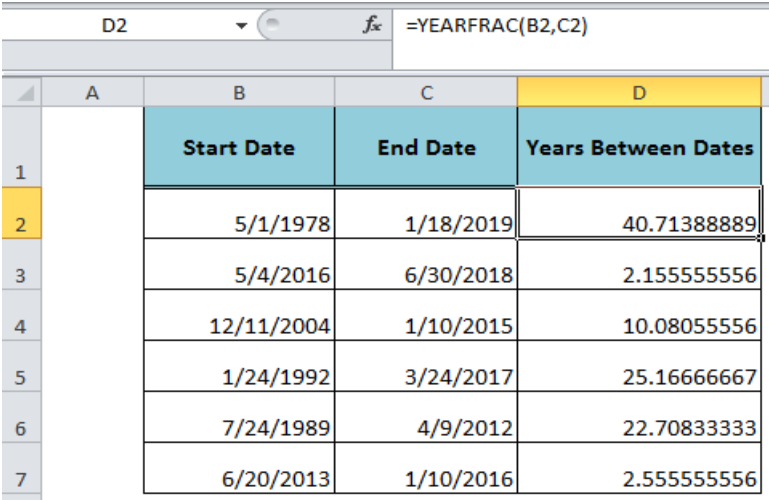Figure 1. Calculating Years Between Dates

## Using YEARFRAC Function to Calculate Years Between Dates

The YEARFRAC function calculates years between two dates, including the fractional value. The decimal number represents the fraction of the year between the dates. The formula to get the difference between dates in terms of years, including fraction, is;

`=YEARFRAC(B2, C2)`

Apply this formula in cell D2 and drag the fill handle to get the other calculations.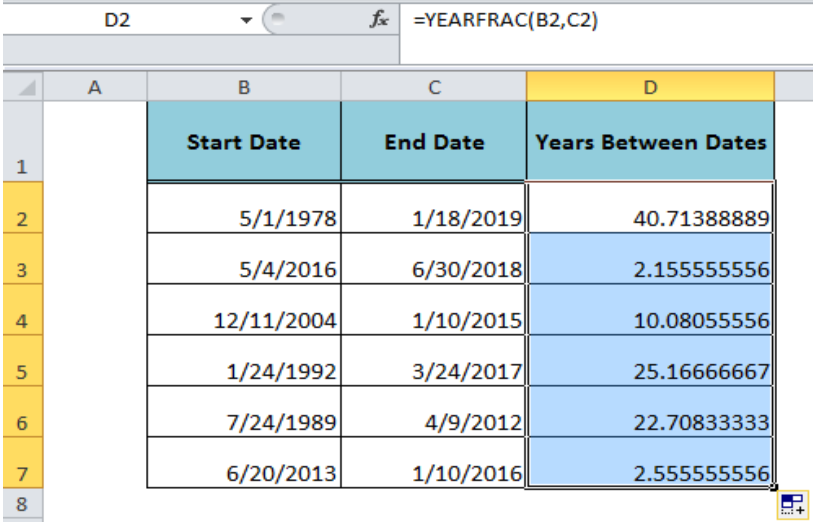Figure 2. Applying the YEARFRAC Function

To round the decimal number or fractional value to nearest whole number we can wrap the above formula in the ROUND function, such as;

`=ROUND(YEARFRAC(B2,C2),0)`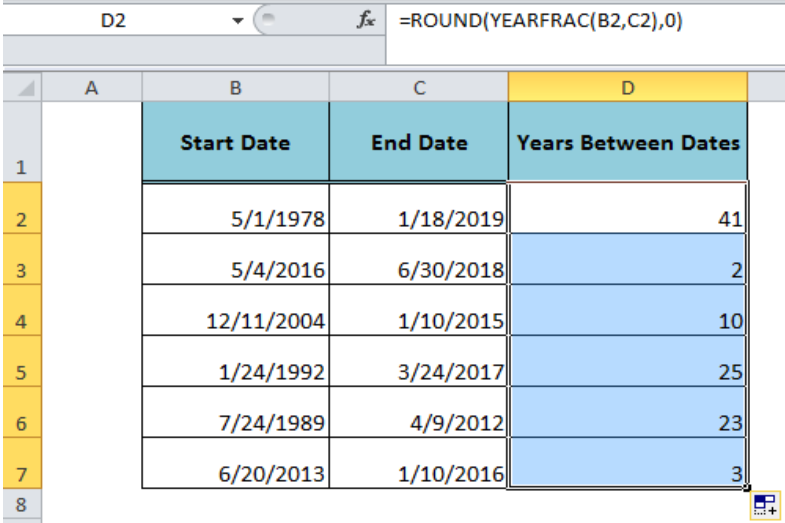Figure 3. Rounding the Fractional Value to Nearest Whole Number

If we want to return only the integer part of the year’s value without fractional value, then we need to wrap the YEARFRAC function inside the INT function, such as;

`=INT(YEARFRAC(B2,C2))`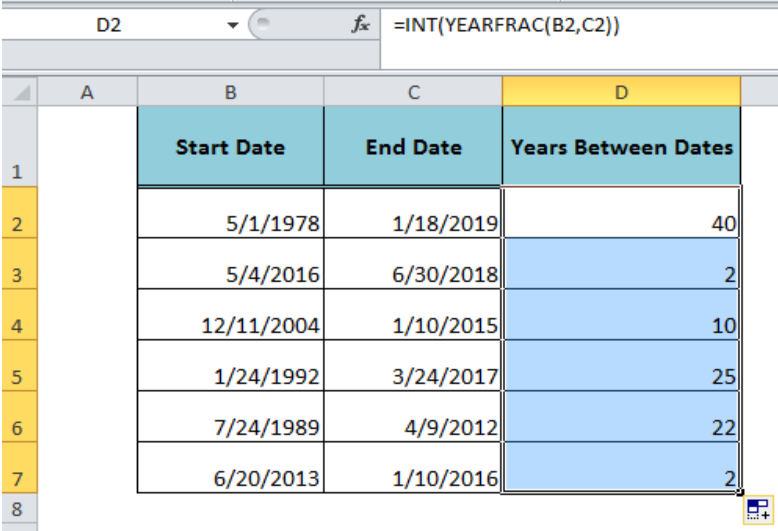Figure 4. Getting the Year Value Without Fractional Value

## Using DATEDIF Function to Calculate Years Between Dates

The DATEDIF function calculates the number of years between two dates when the interval argument is specified as “Y” as per its syntax, such as;

`=DATEDIF( start_date, end_date, “Y” )`

When we apply this function with “Y” interval then it calculates years between two dates a complete calendar year without fractional value. As per our example, we need to apply the following DATEDIF formula in cell D2, and copy or drag down the fill handle to other cells;

`=DATEDIF(B2,C2,"Y")`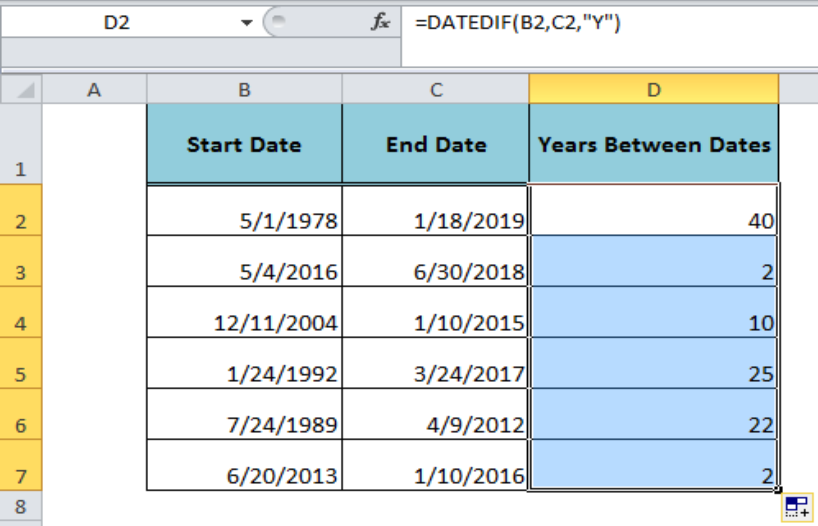Figure 5. Calculating Years Between Dates with DATEDIF Function

## Instant Connection to an Expert through our Excelchat Service

Most of the time, the problem you will need to solve will be more complex than a simple application of a formula or function. If you want to save hours of research and frustration, try our live Excelchat service! Our Excel Experts are available 24/7 to answer any Excel question you may have. We guarantee a connection within 30 seconds and a customized solution within 20 minutes.

### Did this post not answer your question? Get a solution from connecting with the expert.Another blog reader asked this question today on Excelchat:
Solution examplesI need a datedif function that calculates the difference in 2 dates and if it returns more than 5 years, I need it to say yes and if not no.
Solved by O. Y. in 14 minsI have 2 cells containing a % value. The value for both is 9%. I want to conditionally format these cells as duplicate values but excel only highlights them as duplicate values if the decimal point is exactly the same. How can i correct this?
Solved by S. C. in 40 minsHi there. I need a column to automatically calculate the numbers of days from a start time to today's date.
Solved by T. F. in 16 minsi have this formula currently which works but not if I want it for every day - any ideas ?? (NETWORKDAYS.INTL(L1362,M1362,11,BH!A:A)-1)*("17:30"-"8:30")+IF(NETWORKDAYS.INTL(M1362,M1362,11,BH!A:A),MEDIAN(MOD(M1362,1),"8:30","17:30"),"17:30")-MEDIAN(NETWORKDAYS.INTL(L1362,L1362,11,BH!A:A)*MOD(L1362,1),"8:30","17:30")
Solved by A. D. in 28 minsI need help with this formula. I need this to include months =INT((M4)/7)&" weeks"&IF(MOD(M4,7)=0,"",", "&INT(MOD(M4,7))&" days") I have the number of Days to Start with
Solved by F. A. in 29 mins## Subscribe to Excelchat.coAnother blog reader asked this question today on Excelchat: